# Search

About 67 Search Results Matching Types of Worksheet, Worksheet Section, Generator, Generator Section, Kids Sudoku Puzzle3, Subjects matching Math, Grades matching 4th Grade, Page 2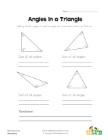## Angles in a Triangle Worksheet

Kids learn that the angles of a triangle always ad...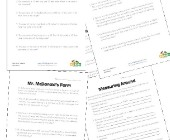## Area and Perimeter Word Problems

This collection of area and perimeter worksheets c...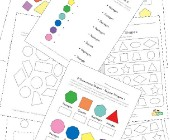## Geometry Worksheets

Help kids learn the early basics of geometry inclu...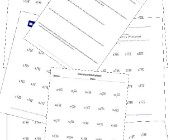## Division Worksheets

Help kids learn division with this collection of m...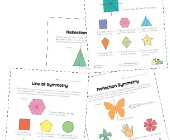## Symmetry Worksheets

Help kids learn about the concept of symmetry with...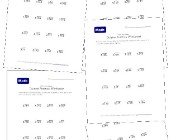## Simple Division Worksheets with Remainders

This section of division worksheets is dedicated t...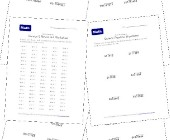## Long Division Worksheets

Help kids practice long division with this set of ...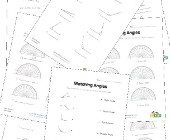## Angles Worksheets

Help kids learn about angles with this collection ...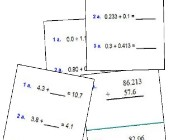Help kids practice decimal addition with the follo...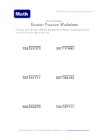## Long Division Worksheet 5

6 hard long division problems for kids to practice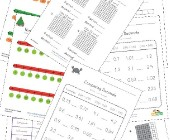## Decimal Worksheets

This collection of free decimal worksheets will he...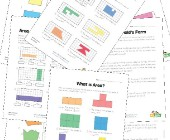## Area and Perimeter Worksheets

Help kids learn to calculate the area and perimete...